New update is available. Click here to update.
Last Updated: Jun 30, 2023

# Dices Problem

## Introduction

The concepts and questions on dice might confuse some people, but it can be easy for people who have a great visual bent of mind and visualization.

We can relate it with cubes with a slightly higher logic and its conceptual application.

Dice is a very important part of the reasoning section of aptitude. The frequency of the questions asked on dice is high every year.

### What is a Dice?

Dice is basically a cube that has different numbers on all its faces. The numbers on faces are usually a single digit, which ranges from 1 to 6.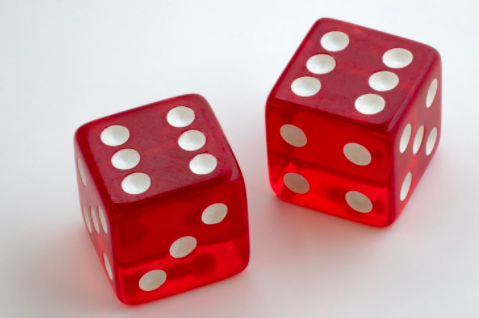### The number on a Die

The way of numbering on a dice is done very specific in nature. It should be kept in mind that the sum of the number on the opposite faces of dice will always be equal to ‘7’. The number 1 will be opposite to number 6 to make the opposite face sum ‘7’; similarly, the number 2 is opposite to 5, and 3 is opposite to 4. Such that:

• A  1+6=7
• B  2+5=7
• C  3+4=7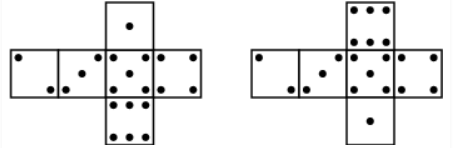Fig: The numbering scheme of dice.

## Classification Of Dice

The base dice is classified majorly into two types.

• Standard dice
• Ordinary dice

Standard Dice: When the given dice are rolled, if the number that appears on the faces of the two dice do not match with each other, then they are called standard dice.

Example: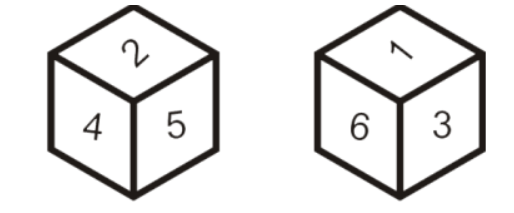Ordinary Dice: When the given dice are rolled, If one or more than one number appears on the faces of dice matches between two dice, it is known as ordinary dice.

Example: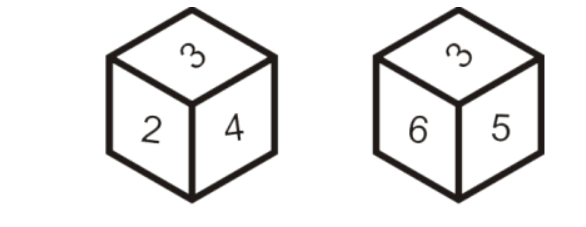In the above-given dice, the number ‘3’ is common for both the dice.

### Open Dice

In the case of open dice, all the six faces of the dice are shown. The dies show the opposite position of rows and columns dice.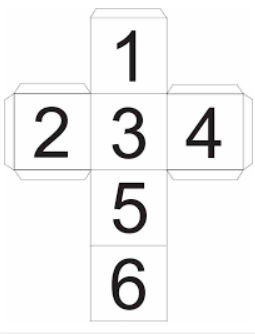The above figure is a schematic representation of the open dice. To explore more on it, you can visit Code Studio.

## Constructed and Deconstructed Dice

### Constructed Dice

This section of dice gives the idea of a constructed version of dice. The questions on this topic will be based on the visualization of the given flattened-out version of dice.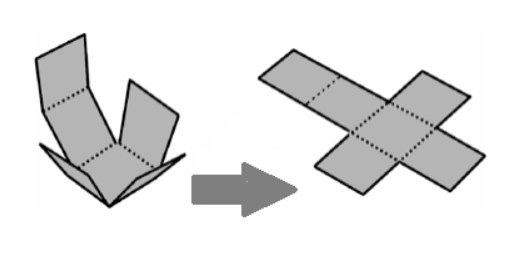Here we have achieved the conversion of constructed dice to flat end die. To explore more on it, you can visit Coding Ninjas Studio.

### Deconstructed Dice

This section of dice gives an idea of the flattened-out version of dice. The questions on this topic will be based on the visualization of what is at the other end of the given constructed dice.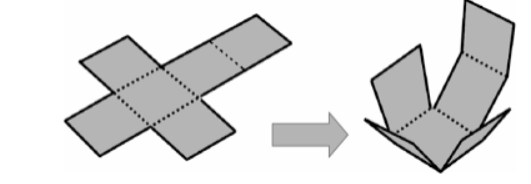Here we convert flat end dice to constructed dice.

Let’s move on to see some important rules about dice.

## Rules to remember

Here we have to remember a few rules so that we can do the problem on the dice topic easily.

Rule 1:

If one of the numbers is common for both the dice that means two dice have the same surface, then the remaining surfaces of both the given dice are opposite each other.

Example: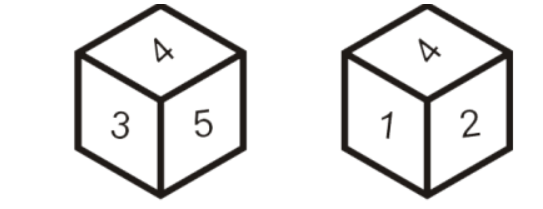In the given two dice, the number 4 is common in both the dice and the numbers 3 and 5 on the first dice, and the number 1 and 2 on the other dice are opposite each other. We can say that the number 3 is opposite 1, and 5 is opposite to 2.

Rule 2:

If any two numbers of dice are the same in the given two dice, irrespective of their position then we can conclude that the remaining third number on the dice, in both the dice, are opposite to each other.

Example: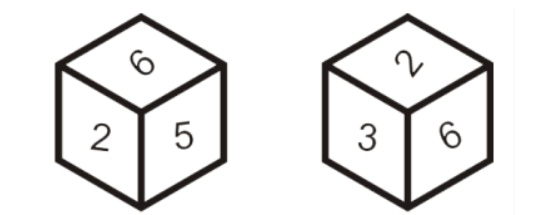We can clearly see in the above scenario, irrespective of their place or position in the given two dice that the numbers 6 and 2 are common for both the dice. This means the third number 5 on the first dice and the number 3 on the second dice are opposite.

Rule 3:

If there is one element common that is common on both the dice at different positions, then we have to rotate the dice in a clockwise direction so that we can get the opposite surface.

Example: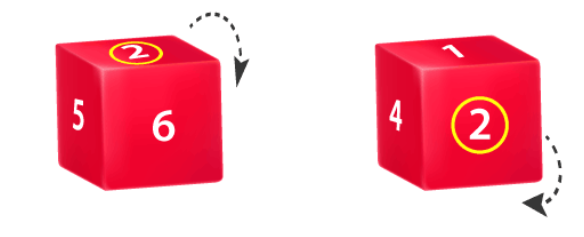From the figure, we can see that the number ‘2’ is common for both the dice. At the same time, it is occurring at a different position. To find out which number is appearing on the opposite face, we have to rotate the die in a clockwise direction in such a way that the common number is the reference.

## Observations of an Open Die

The below-given figure is a flat-end version of a dice.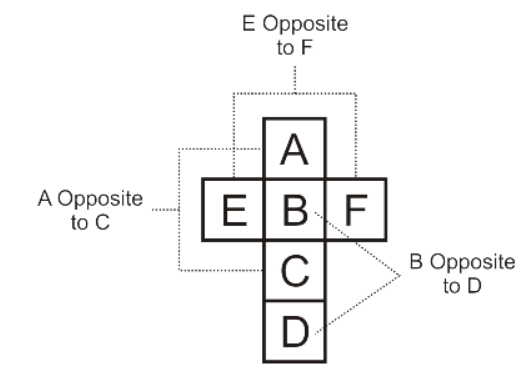In the above figure the faces B & D, E & F, A & C are opposite to each other and their surfaces will never touch each other.

## Example Problems

Example 1:

Find the possible combinations of the die. Given die is an open die.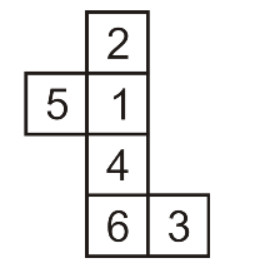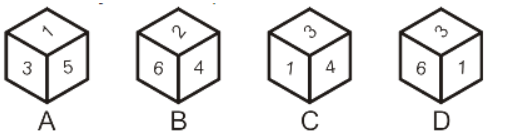Explanation:

Option 1 is not possible because  3 cannot be opposite to 5.

The second option is also wrong because 2 cannot be adjacent to 4.

And option 4 is also not possible as 1 is not adjacent to 6.

So, option 3 is the only possible combination.

Hence the correct answer is option 3.

Example 2

Select the die that is similar to the die-formed from the open die.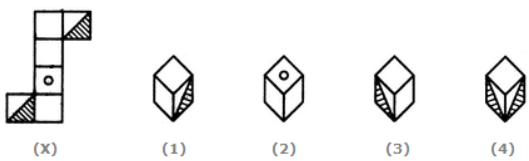Explanation:

In the above figure, option 1 is possible as the top face of the cube is shaded, and the adjacent faces of the cube are blank.

Option 2 is not possible as the surface with a dot on the cube should lie between the shaded one, but in the figure, we have a blank.

Option 3 is possible, but the 4th one is impossible because the two shaded faces of the cube cannot be adjacent to each other.

Thus, the correct answer is option C.

### What is a dice?

Dice is a cube with each face having numbers in the range of 1 to 6.

### What is an important fact about the opposite side of a die?

The Sum of numbers on the opposite face of dice is always 7.

1. Standard Die

2. Ordinary Die

## Conclusion

Aptitude is an important aspect for preparations for placements or when applying for any other jobs and interviews. It is important to have a good handle on Aptitude questions to ace those. In this blog, we discussed one of the important topics of aptitude known as the dice problem. Along with that, we’ve also discussed the various conditions and various rules that come in handy whilst solving the problems.